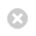Close

Gatsby Computational Neuroscience Unit

HomeArnak Dalalyan (ENSAE ParisTech)

Wednesday 4th March 2020

Time: 4pm

Ground Floor Seminar Room

25 Howland Street, London, W1T 4JG

All-In-One Robust Estimator of the Gaussian Mean (arXiv:2002.01432)

The goal of this talk is to show that a single robust estimator of the mean of a multivariate Gaussian distribution can enjoy five desirable properties. First, it is computationally tractable in the sense that it can be computed in a time which is at most polynomial in dimension, sample size and the logarithm of the inverse of the contamination rate. Second, it is equivariant by translations and orthogonal transformations. Third, it has a high breakdown point equal to 0.5, and a nearly-minimax-rate-breakdown point approximately equal to 0.28. Fourth, it is minimax rate optimal when data consist of independent observations corrupted by adversarially chosen outliers. Fifth, it is asymptotically optimal when the rate of contamination tends to zero. The estimator is obtained by an iterative reweighting approach. Each sample point is assigned a weight that is iteratively updated using a convex optimization problem. We also establish a dimension-free non-asymptotic risk bound for the expected error of the proposed estimator. It is the first of this kind results in the literature and involves only the effective rank of the covariance matrix.

(Joint work with Arshak Minasyan)